# MCAT Physical : Reaction Rate and Rate Laws

## Example Questions

2 Next →

### Example Question #91 : Physical Chemistry

A scientist is studying a reaction, and places the reactants in a beaker at room temperature. The reaction progresses, and she analyzes the products via NMR. Based on the NMR readout, she determines the reaction proceeds as follows: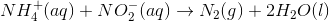In an attempt to better understand the reaction process, she varies the concentrations of the reactants and studies how the rate of the reaction changes. The table below shows the reaction concentrations as she makes modifications in three experimental trials.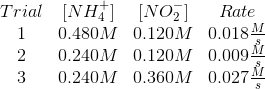Which of the following most closely approximates the rate law for this reaction?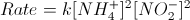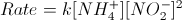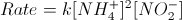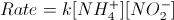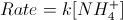Explanation:

The reaction table in the passage indicates that the reaction rate varies in a 1-to-1 fashion as you vary the each reactant, while holding the other constant.

The rate law is written as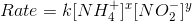.

Compare trials 1 and 2 to see that doubling the ammonium concentration doubles the rate. The reaction is first order for ammonium:.

Compare trials 2 and 3 to see that tripling nitrate concentration triples the rate. The reaction is first order for nitrate: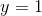.

The final rate law is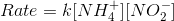.

### Example Question #91 : Physical Chemistry

The rate for a reaction is given by the equation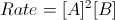. What is the overall order of the reaction?

2

1

5

3

4

3

Explanation:

To find the overall order of a reaction, look at the exponents for the reaction rate law. Since A has an exponent of two and B has an exponent of one, the overall reaction has an order of three.

### Example Question #11 : Reaction Rate And Rate Laws

A first-order reaction has a rate constant: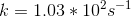How long does it take for the concentration of the reactant to reach one-fourth of its original amount?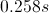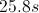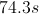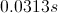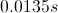Explanation:

For a first order reaction, use the equation: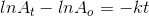In this formula,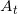is the concentration at a given time,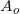is the initial concentration,is the rate constant, andis the time elapsed.

If we assume the initial concentration to be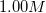, then we can use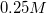as the final concentration. Using these values and the given rate constant, we can calculate the time.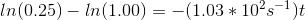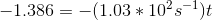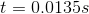### Example Question #13 : Reaction Rate And Rate Laws

A kinetics experiment is constructed, and the following rates of product formation are observed after adding various substrates. The results are recorded below. What is the equation for the rate law?

 A B C Initial Rate (M/s) 0.1M 0.2M 0.2M 1.5 0.2M 0.2M 0.2M 3 0.2M 0.2M 0.4M 3 0.2M 0.4M 0.2M 12

Rate = k[A][B]

Rate = k[A][B]2

Rate = k[A]2[B]

Rate = k[A][B][C]

Rate = k[A][B]2

Explanation:

We can look at the differences between trial 1 and trial 2 to see that the reaction is first order with respect to [A], since the rate doubles when the reactant concentration doubles. Reactants are considered first order when this 1:1 relationship between concentration and rate is observed.

We can look at trials 2 and 3 to see that [C] has no effect on the reaction, making it a zeroth order reactant and simply equal to 1 in the rate law.

Trials 3 and 4 show that when [B] doubles, the rate quadruples. This means it is second order with respect to [B], increasing the rate by the square of the increase in concentration. Since we already know that reactant C does not affect the rate, it is irrelevant that its concentration is halved.

This gives us Rate = k[A][B]2.

2 Next →

### All MCAT Physical Resources